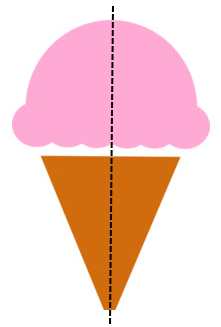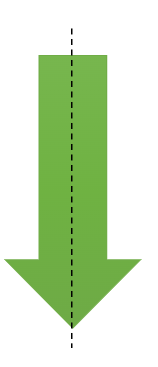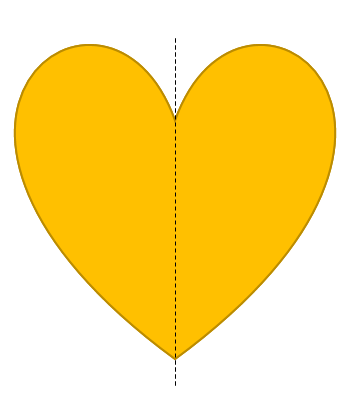# Math > Year 2

## Vertical lines of symmetry

Symmetry
Symmetry is when an object looks the exact same on one side as the other.Lines of Symmetry

A line of symmetry is a line that cuts a shape exactly in half.If you fold the shape along the line, the two halves will be the same. Similarly, the shape would not alter if a mirror was placed along the line.

Vertical Line

A vertical line is one that goes up and down.Vertical Lines of symmetry
A vertical line of symmetry is a line from up to down that cuts a shape exactly into two same halves.

Example 1Here, the vertical line divides the shape into two identical parts.

Hence, it is a vertical line of symmetry.

Example 2Here, the vertical line divides the shape into two identical parts.

Hence, it is a vertical line of symmetry.

//